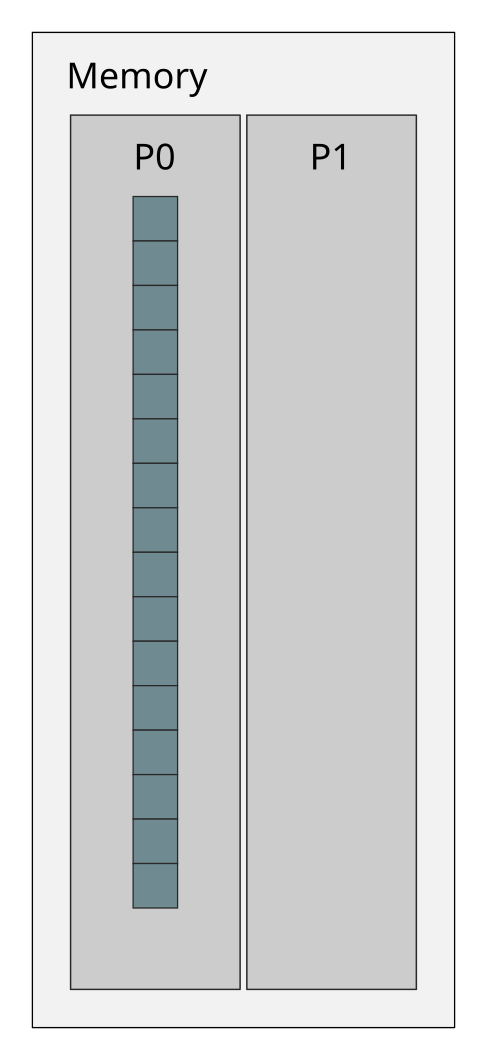## Want to keep learning?

This content is taken from the Partnership for Advanced Computing in Europe (PRACE)'s online course, Python in High Performance Computing. Join the course to learn more.
4.9

# Case study: Parallel sum

How can a problem be split into smaller pieces that can be executed in parallel? How to do it efficiently? These are the key questions when designing a parallel algorithm.

Designing a good strategy for work distribution is usually the most essential part of writing a parallel program. Common approaches utilise either data parallelism or task parallelism. In data parallelism one distributes a (large) dataset to multiple processors and then the processors operate on separate pieces of the data in parallel. In task parallelism one breaks down the algorithm into smaller tasks that are then distributed and executed in parallel.

Let us now look into an example implementation of a parallel algorithm for calculating the sum of all values in an array.

## Initial state

Assume we have an array A that contains a large number of floating point numbers. The values have been read from a file by the first MPI task (rank 0).## Goal

Calculate the total sum of all elements in array A in parallel.

## Parallel algorithm

Our parallel algorithm consist of four main steps: 1) distribute the data, 2) compute local sums, 3) gather the partial results, and 4) compute the total sum. Steps 1 and 3 can be further broken down into sub-steps to better illustrate the MPI communication needed.

1. Scatter the data
1.2. send operation for scatter
2. Compute partial sums in parallel
3. Gather the partial sums
3.2. send operation for gather
4. Compute the total sum


### Step 1.1: Receive operation for scatter### Step 1.2: Send operation for scatter### Step 2: Compute partial sums in parallel### Step 3.1: Receive operation for gather### Step 3.2: Send operation for gather### Step 4: Compute the total sumIn the next activities you will learn how to carry out the above operations in practice.